# Pressure Conversion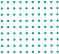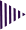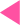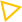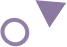=
Pressure Conversion Calculator

# Online pressure conversion calculator

Pressure is a scalar quality reflecting how force acts on a surface.
P = F/A
Where P is equal to pressure, F is the normal force and A is area. Several units for pressure directly relate force to area because pressure is derived from force and area.

A pound per square inch (PSI) is the imperial measurement of pressure. Pressure in PSI is commonly measured as gas pressure (pneumatic pressure) or liquid pressure (hydraulic pressure).
The pound per square inch, or pound-force per square inch, is an avoirdupois unit of pressure or stress. An inch square area is subjected to one pound's force applied to a one square inch area. The SI unit of 1 psi is approximately 6895 Pa

### Pressure Conversion Units Definitions

• Pound per square (PSI) converter definition:-

A pound per square inch (PSI) is the imperial measurement of pressure. Pressure in PSI is commonly measured as gas pressure (pneumatic pressure) or liquid pressure (hydraulic pressure).
The pound per square inch, or pound-force per square inch, is an avoirdupois unit of pressure or stress. An inch square area is subjected to one pound's force applied to a one square inch area. The SI unit of 1 psi is approximately 6895 Pa

• Pascals converter definition:-

Pascals are equivalent to one newton per square metre, or one kilogram per metre per second squared in SI units. The kilopaccal (kPa) of 1,000 newtons per square metre is more commonly used since this unit is too small for many purposes.
Pascals are SI-derived units of pressure that are used to measure internal pressure, stress, Young's modulus, and ultimate tensile strength. Pascal's unit is equal to one newton per square metre in the CGS system and is named after Blaise Pascal

• Torrs converter definition:-

- Torrs are units of pressure based on absolute scales, defined as 1/760 of a standard atmosphere. Therefore, one torr equals 101325/760 pascals. Historically, one torr was equivalent to one millimeter of mercury, but subsequent definitions made them slightly different.
A unit of pressure equivalent to 1 mm of mercury in a barometer and 133.32 pascals.
Torrs are units of pressure based on an absolute scale, equal to 1/760 of a standard atmosphere. Hence, one torr equals 101325/760 pascals. Historically, one torr was the same as one millimeter of mercury, but later redefinitions made them slightly different

• Bar converter definition:-

The bar is a metric unit of pressure, but it is not part of the International System of Units. This is defined as being equal to 100,000 Pa, which is slightly less than the current average atmospheric pressure on Earth at sea level.
It is a horizontal line that is written above a mathematical symbol to give it some special meaning. Macrons are sometimes used to describe bars in front of single symbols, such as (voiced "-bar").